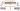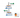# Speed Time and Distance Formula

Hello all,

In this Blog we will discuss one of the crucial topic from which two to three questions may asked in almost all competitive exams i .e. Speed, time and distance.SOME BASIC RULES:

Speed: – The rate at which a particular distance is covered by an object in motion. The unit of speed may be m/s, km/hr, km /min or km/day.

Distance: – It refers to the extent of space between two points. The unit of distance is meters (m), kilometres (km) and centimetres (cm).

Time: – It refers to an interval separating two events. The unit of time is seconds (sec or s), minutes (min), and hours (hr).

The basic concept of speed, time and distance is the relation between these three variables. The speed of a body is the distance covered by body per unit time.

• Speed = Distance/time.
• Time = Distance/ Speed.
• Distance = Speed × Time.
• If speed is constant, distance and time are directly proportional.
• If time is constant, speed and distance are directly proportional.
• Speed and time is inversely proportional. When distance is constant.

CONVERSION OF UNITS

• If the speed is given in km per hour and we want to convert it in to meter per second, we have to multiply the given speed by 5/18.

1km/hr = 5/18 m/s

E.g. 36 km/hr = 36×5/18 = 10 km/hr.

• If the speed is given in meter per second and we want to convert it in to km per hour, we have to multiply the given speed by 18/5.

1m/s = 18/5 km/hr

E.g. 10 km/hr = 10×18/5 =36 km/hr.

Question 1) A person crosses a street 600m long in 5 minutes. His speed in km/hr is?

By using formula: S=D/T

5 min = 5×60 sec = 300 sec

Speed of man = 600/300 m/sec

= 2 m/sec

1 m/sec = 18/5 km/hr

2×18/5 = 36/5 km/hr

= 7.2 km/hr

Question 2) A train 400m long runs at 30 m/s find time?

S= 30m/s

T=?

By using formula T=D/S

T= 400/30

T= 13.3 sec.

Question 3) Ram walks 325m every day. How many kilometres will he walk in four weeks?

Answer: In one week there are 7 days

Therefore, in four week = 4×7 = 28 days

=28×325= 9100m

To convert in kilometre we have to divide it by 1000

=9100/1000= 9.1 km.

Concept of Average Speed:

• If a person travels certain distance with x km/hr and same distance with y km/hr then average speed is = 2xy/x+y.
• In case a person travels one-third of distance at speed of x km/hr, the next one-third at speed of y km/hr and the last one-third distance z km/hr, then the average speed of person is = 3xyz/xy+yz+zx.
• If the ratio of speeds of A and B is a:b then the ratio of time taken by them to cover same distance is 1/a: 1/b or b: a.
• If two quantities are inversely proportional then if one of them increase by (a/b)th then other quantity decreases by (a/a+b)th

Question 4) A man covers a certain distance by car at 60 km/hr and returns back to starting point riding on scooter at 100 km/hr. Find his average speed for whole journey.

Average speed = 2xy/x+y

Average speed = (2×60×100)/ (60 +100) km/hr

= (2×60×100)/160 km/hr

= 75 km/hr.

Question 5) Ram travels one-third of distance at speed of 20 km/hr, the next one-third at speed of 30 km/hr and the last one-third distance 40 km/hr, then find the average speed he covers during journey?

Average speed = 3xyz/xy+yz+zx

= 3×20×30×40/ 20×30+30×40+40×20

= 3×20×30×40/600+1200+800

=3×20×30×40/2600

=27.69 km/hr.

Question 6) If speed increases by 20% then find the percentage decrease in time to cover same distance?

By percentage parameters, we know that 20 % is 1/5.

Therefore, 20% = 1/5 = a/b

Hence a=1, b= 5

Hence, time decreases by (a/a+b)th = 1/(1+5) =1/6th

% decrease = 1/6 × 100 = 16.66 %

Question 7) A women travel a certain distance between his home and office on scooter. With an average speed of 30 km/hr he is late by 15 min. However with a speed of 40 km/hr he reaches 5 min earlier. Find the distance between his home and office.

Let the distance be x km.

Time taken to cover x km at 30 km/h = x/30 hr

Time taken to cover x km at 40 km/h = x/40 hr

Difference between the times = 15 min= 15/60 hr =1/4 hr

= x/30 – x/40 = ¼

= 40x-30x/40×30 = ¼

= 10x/40×30 =1/4

= 40x = 40×30

x = 40×30/40

x = 30.

x = 30 km

Question 8) Walking ¾ of his usual speed, a person is 20 min late to his office. Find his usual time to cover the distance?

Answer: Let the usual speed of person be s

We know that s=d /t or d/s = t

d/ (3s/4) – d/s = 20

4d/3s – d/s = 20

4ds-3ds/3s2 = 20

ds / 3s2 = 20

By cancelling s

d/s =20 × 3 = 60 min.

Applications of Speed, Time and Distance:-

PROBLEMS ON TRAINS

• When a train crosses a man/pole/tree the distance is train’s own length

S = L/t (L = Distance)

• When a train crosses a bridge/tunnel/platform, then distance is train’s length + length of bridge/tunnel/platform.

S = Lt + Lp/t       (Lt = Length of train, Lp= Length of platform)

## CONCEPT OF RELATIVE SPEED• When two objects or trains move in the opposite direction, then relative speed is equal to the sum of their speeds.

Relative speed = (A+B) Km/hr

• When two objects or trains move in the same direction, then relative speed is equal to the difference between their speeds.

Relative speed = (A-B) Km/hr

Question 9) Two trains of length 80 m and 90 m are moving in opposite directions at 10 m/s and 7m/s respectively. Find the time taken by the trains to completely cross each other.

Answer:  By using formula T = D/S where s is relative speed i.e. A+B

Required time = (80+90)/ (10+7) sec

= 170/17 sec

= 10 sec.

Question 10) Two trains of length 85 m and 90 m are moving in same directions at 9 m/s and 8m/s respectively. After how much time both the trains cross each other completely?

T= length of 1st train + length of 2nd train

1st train speed – 2nd train speed

Required time = (85+90)/ (9-8) sec

= 175/1 = 175sec

Question 11) A train passes a standing man in 6 sec and a 210 m long platform in 16 sec. Find the length and speed of the train.

S = Lt + Lp/t     (Lt = Length of train, Lp= Length of platform)

Let the length of train be L and speed be x m/sec.

L/6 = x

Also, (L+210)/16 =x

= L/6 = (L+210)/16

= 16 × (L) = 6× (L+210) (By cross multiplying)

= 16 L = 6L + 1260

= 16L-6L = 1260

= 10 L = 1260

L =1260/10

L= 126 m

Hence,

Speed = x = L/6 =21 m/sec.

Question 12) Two trains of equal lengths take 5 sec and 6 sec to cross a pole respectively. If two trains are moving in the same direction, then how much time will they take to cross each other completely?

Answer:   Let the length of the trains be L metre each.     (By using s=d/t)

Speed of train1= L/5 m/s

Speed of train 2= L/6 m/s

Time taken to cross each other = Sum of their lengths/ relative speed.

= L+L/ (L/5-L/6)

= 2L / (6L-5L)/30

= 2L/ (L/30) = 60 sec

## BOATS AND STREAMS.In case of boat related problems, Boat speed in still water is U km/hr and Stream speed is V km/hr then:-

• If the flow of stream and boat are in opposite direction then Speed is known as Upstream = (U-V) km/hr.
• If the flow of stream and boat are in same direction then Speed is known as Downstream = (U+V) km/hr.
• The speed of boat in still water = ½ (Downstream + Upstream).
• The speed/Rate/Velocity of stream/current = ½ (Downstream – Upstream).

Question 13) A man can row upstream at 10 km/hr and downstream at 20km/hr. Find the man’s rate in still water and speed of the stream.

Answer: The speed of boat in still water = ½ (Downstream + Upstream).

=1/2 (20+10)

= 30/2

= 15km/hr

The speed/ Rate of stream =  ½ (Downstream – Upstream)

= ½ (20 -10)

= 10/2

= 5km/hr.

Question 14) The speed of boat in still water is 4 km/hr. The speed of river is 1.5 km/hr. The boat starts travelling from point A to point B upstream and then come downstream, the distance between the points is 20 km. In what time can the boat travel the started distance?

Answer: Sb = 4km/hr Sr=1.5km/hr      (Sb = speed of boat, Sr=speed of river)

Speed downstream = (Sb+ Sr)

= (4 +1.5)

=5.5 km/hr

Speed upstream = (Sb– Sr)

= (4 -1.5)

= 2.5 km/hr

20/2.5 + 20/5.5 = T         (T =D/S)

By solving 200/25+200/55 = T

8+ 40/11 = T

88+ 40 =   128

11            11

= 11.63 hours.

Question 15) In one hour, a boat goes 12 km along the stream and 6 km against it. Find the speed of boat in still water?

Answer :  Let, us suppose the speed of boat in still water = x km/hr .

The Speed of stream = y km/hr

By using T= D/S

= 12/x+y =1

= x+y =12………………………………… (1)

= 6/x-y = 1

=x-y =6 ………………………………….(2)

By solving equation (1) and (2)

x + y =12

x – y =  6

–      –      –

2y   =  6

y = 6/3 = 2 km/hr

substuting the value in equation (1 )

x + y = 12

x + 2 =12

x = 12 – 2 = 10 km/hr.